# Algebra II : Geometric Sequences

## Example Questions

1 3 Next →

### Example Question #21 : Mathematical Relationships And Basic Graphs

A geometric sequence begins as follows: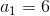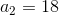Which of the following gives the definition of itsth term?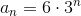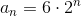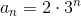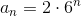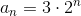Explanation:

Theth term of a geometric sequence is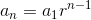where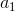is its initial term andis the common ratio between the terms.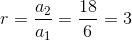Substituting, the expression becomes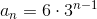By factoring and remultiplying, this becomes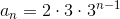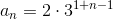### Example Question #21 : Geometric Sequences

A sequence begins as follows: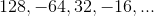Which statement is true?

The sequence may be geometric.

The sequence may be arithmetic.

None of these

The sequence cannot be arithmetic or geometric.

The sequence may be arithmetic and geometric.

The sequence may be geometric.

Explanation:

An arithmetic sequence is one in which each term is generated by adding the same number - the common difference - to the previous term. As can be seen here, the difference between each term and the previous term varies from term to term: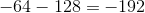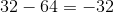The sequence cannot be arithmetic.

A geometric sequence is one in which each term is generated by multiplying the previous term by the same number - the common ratio. As can be seen here, the ratio of each term to the previous one is the same: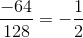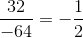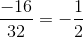The sequence could be geometric.

1 3 Next →

### All Algebra II Resources